Courses

# Sequential Logic Circuits - 1

## 10 Questions MCQ Test Mock Tests Electronics & Communication Engineering GATE 2020 | Sequential Logic Circuits - 1

Description
This mock test of Sequential Logic Circuits - 1 for GATE helps you for every GATE entrance exam. This contains 10 Multiple Choice Questions for GATE Sequential Logic Circuits - 1 (mcq) to study with solutions a complete question bank. The solved questions answers in this Sequential Logic Circuits - 1 quiz give you a good mix of easy questions and tough questions. GATE students definitely take this Sequential Logic Circuits - 1 exercise for a better result in the exam. You can find other Sequential Logic Circuits - 1 extra questions, long questions & short questions for GATE on EduRev as well by searching above.
QUESTION: 1

### For which of the following flip-flops, the output is clearly defined for all combinations of two inputs?

Solution:

For J-K flip-flop, the output is clearly defined for all combinations of two inputs. For S-R flip-flop output is not defined when S = R = 1. A D flip-flop has only one input.

QUESTION: 2

### What J-K input condition will always set ‘Q+ upon the occurrence of the active clock transition ?

Solution:

Truth table for J-K flip-flop is shown below.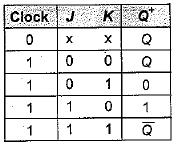When J = 1 and K = 0, output (Q+) is always set upon the occurrence of the active clock transition.

QUESTION: 3

### In a J-K flip-flip, toggle means

Solution:

In a J-K flip-flop, toggle means change the output to the opposite state. A J-K flip-flop toggles when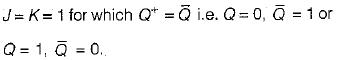QUESTION: 4

The output of S-R flip-flop when S = 1, R = 0 is

Solution: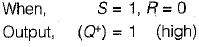QUESTION: 5

An eight stage ripple counter uses a flip-flop with propagation delay of 75 nano-seconds. The pulse width of the strobe is 50 nano-seconds. The frequency of the input signal which can be used for proper operation of the counter is approximately equal to

Solution:

Maximum time taken for all flip-flops to stabilize is (75 ns x 8) + 50 ns = 650 ns.
Frequency of operation must be less than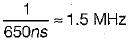Thus, option (d) is correct.

QUESTION: 6

The output of a J-K flip-flop with asynchronous preset and clear inputs if ‘1 ’. The output can be changed to ‘0’ with which one of the following conditions?

Solution:

When J = 1, K = 1 and the clock, next state will be complement of the present state. Thus,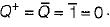QUESTION: 7

A J-K flip-flop can be implemented using D flip- flop connected such that

Solution:
QUESTION: 8

Assertion (A): In general, asynchronous circuits are considerably faster than synchronous circuits.
Reason (R): In an asynchronous circuit, events can occur after one event is completed and there is no need to wait for a clock pulse.

Solution:
QUESTION: 9

Consider the following statements:
1. Sequential circuits are always faster than combination circuits.
2. In an asynchronous circuit there is no problem of stability.
3. The logic circuits whose outputs at any instant of time depend only on the input signals present at that time are known as combinational circuits.
4. In a combinational circuit, for a change in the input, the output appears immediately.
5. In a sequential circuits, the output signals are fed back to the input side.

Which of the statement given above are correct?

Solution:

• Combinational circuits are often faster than sequential circuits since the combinations circuits do not require memory whereas the sequential circuits need memory devices to perform their operations in sequence. Hence statement - 1 is not correct.
• in an asynchronous circuit, events are allowed to occur without any synchronisation In such a case, the system become: unstable which results in difficulties. Hence statement-2 is not correct.
• Statement-3 is correct which is the definitioi of a combinational circuit.
• in a combinational circuit, for a change if the input, the output appears immediately except for the propagation delay througt circuit gates. Thus, statement-4 is no correct.
• In a sequential circuit, an output signal is e function of the present input signals and e sequence of the past input signals i.e. the past output signals since the output signals are fed back to the input side. Hence, statement-5 is correct.
Thus, statements 3 and 5 are only correct.

QUESTION: 10

Assertion (A): A latch is a memory device with the capability of storing one binary digit of information.
Reason (R): A basic latch is made up of cross coupled inverters.

Solution:

A latch is memory device with the capability of storing one binary digit of information because the latch output will remain set/reset until the trigger pulse is given to change the state.
A basic latch is made up of cross coupled inverters as shown below.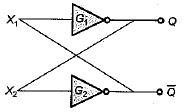Thus, both assertion and reason are true but reason is not the correct explanation of assertion.